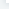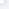# NumPy IO

NumPy IONumpy 可以读写磁盘上的文本数据或二进制数据。NumPy 为 ndarray 对象引入了一个简单的文件格式：npy。npy 文件用于存储重建 ndarray 所需的数据、图形、dtype 和其他信息。常用的 IO 函数有：load() 和 save() 函数是读写文件数组数据的两个主要函数，默认情况下，数组是以未压缩的原始二进制格式保存在扩展名为 .npy 的文件中。savze() 函数用于将多个数组写入文件，默认情况下，数组是以未压缩的原始二进制格式保存在扩展名为 .npz 的文件中。loadtxt() 和 savetxt() 函数处理正常的文本文件(.txt 等)numpy.save()numpy.save() 函数将数组保存到以 .npy 为扩展名的文件中。numpy.save(file, arr, allow_pickle=True, fix_imports=True)参数说明：file：要保存的文件，扩展名为 .npy，如果文件路径末尾没有扩展名 .npy，该扩展名会被自动加上。arr:

# 保存到 outfile.npy 文件上np.save('outfile.npy',a)

# 保存到 outfile2.npy 文件上，如果文件路径末尾没有扩展名 .npy，该扩展名会被自动加上np.save('outfile2',a)我们可以查看文件内容：\$ cat outfile.npy ?NUMPYv{'descr':

'<i8', 'fortran_order':

False, 'shape':

(5,), } \$ cat outfile2.npy ?NUMPYv{'descr':

'<i8', 'fortran_order':

False, 'shape':

(5,), } 可以看出文件是乱码的，因为它们是 Numpy 专用的二进制格式后的数据。我们可以使用 load() 函数来读取数据就可以正常显示了：实例import numpy as npb = np.load('outfile.npy')print (b)输出结果为：[1 2 3 4 5]np.saveznumpy.savez() 函数将多个数组保存到以 npz 为扩展名的文件中。numpy.savez(file, *args, **kwds)参数说明：file：要保存的文件，扩展名为 .npz，如果文件路径末尾没有扩展名 .npz，该扩展名会被自动加上。args:

# c 使用了关键字参数 sin_arraynp.savez("k88.npz", a, b, sin_array = c)r = np.load("k88.npz")print(r.files)

# 查看各个数组名称print(r["arr_0"])

# 数组 aprint(r["arr_1"])

# 数组 bprint(r["sin_array"])

# 数组 c输出结果为：['sin_array', 'arr_0', 'arr_1'][[1 2 3] [4 5 6]][0. 0.1 0.2 0.3 0.4 0.5 0.6 0.7 0.8 0.9][0. 0.09983342 0.19866933 0.29552021 0.38941834 0.47942554 0.56464247 0.64421769 0.71735609 0.78332691]savetxt() savetxt() 函数是以简单的文本文件格式存储数据，对应的使用 loadtxt() 函数来获取数据。np.loadtxt(FILENAME, dtype=int, delimiter=' ')np.savetxt(FILENAME, a, fmt="%d", delimiter=",")参数 delimiter 可以指定各种分隔符、针对特定列的转换器函数、需要跳过的行数等。实例import numpy as npa = np.array([1,2,3,4,5])np.savetxt('out.txt',a)b = np.loadtxt('out.txt')print(b)输出结果为：[1. 2. 3. 4. 5.]使用 delimiter 参数：实例import numpy as npa=np.arange(0,10,0.5).reshape(4,-1)np.savetxt("out.txt",a,fmt="%d",delimiter=",")

# load 时也要指定为逗号分隔print(b)输出结果为：[[0. 0. 1. 1. 2.] [2. 3. 3. 4. 4.] [5. 5. 6. 6. 7.] [7. 8. 8. 9. 9.]]

NumPy IO

## 相关文章